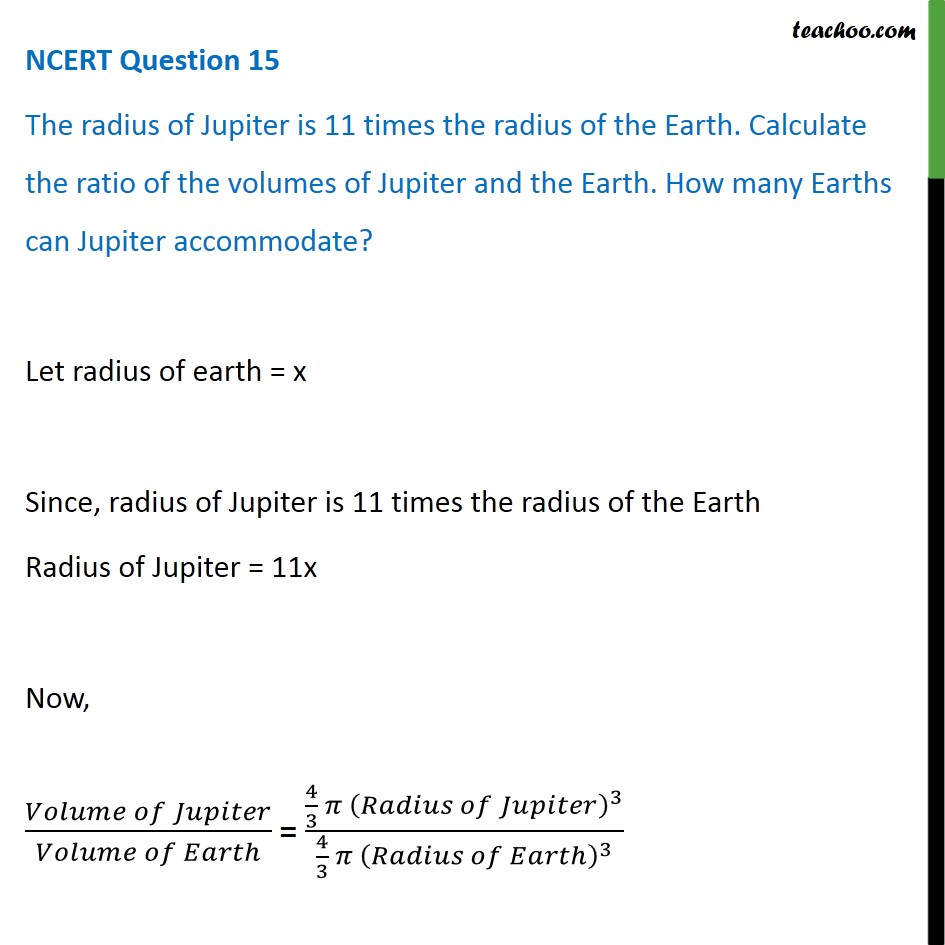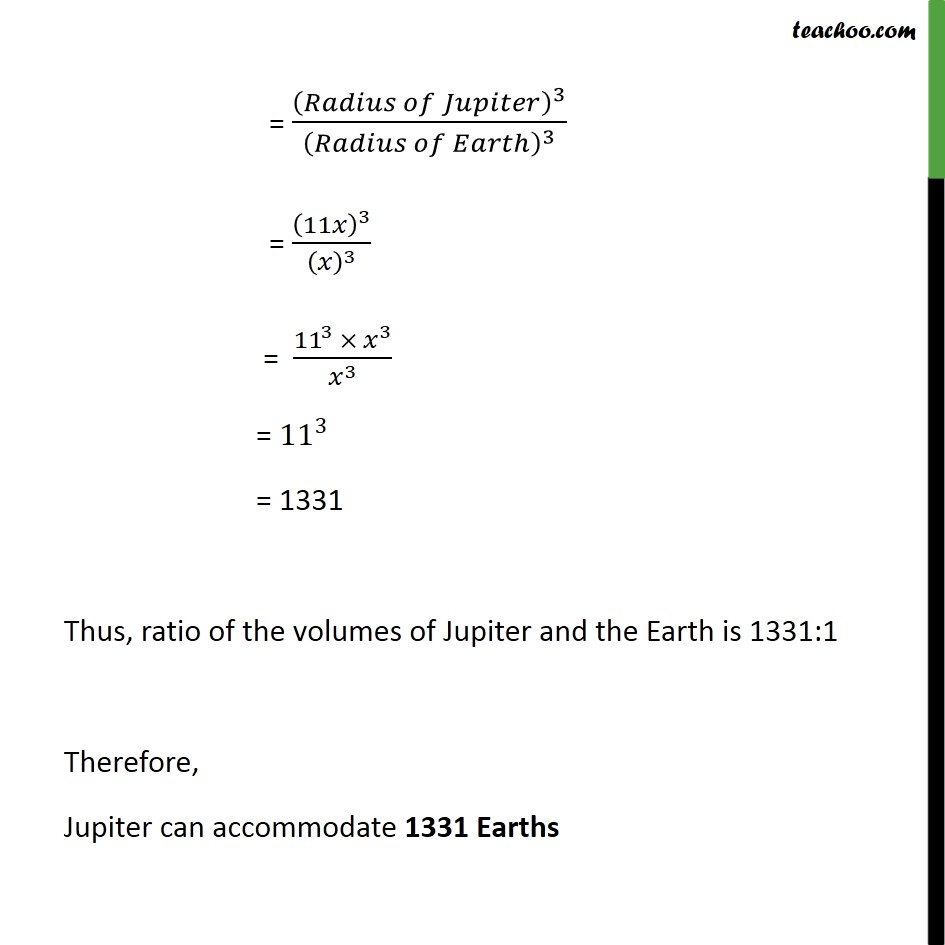NCERT Questions

Class 8
Chapter 17 Class 8 - Stars and the Solar SystemLearn in your speed, with individual attention - Teachoo Maths 1-on-1 Class

### Transcript

NCERT Question 15 The radius of Jupiter is 11 times the radius of the Earth. Calculate the ratio of the volumes of Jupiter and the Earth. How many Earths can Jupiter accommodate? Let radius of earth = x Since, radius of Jupiter is 11 times the radius of the Earth Radius of Jupiter = 11x Now, (𝑉𝑜𝑙𝑢𝑚𝑒 𝑜𝑓 𝐽𝑢𝑝𝑖𝑡𝑒𝑟)/(𝑉𝑜𝑙𝑢𝑚𝑒 𝑜𝑓 𝐸𝑎𝑟𝑡ℎ) = (4/3 𝜋 (𝑅𝑎𝑑𝑖𝑢𝑠 𝑜𝑓 𝐽𝑢𝑝𝑖𝑡𝑒𝑟)^3)/(4/3 𝜋 (𝑅𝑎𝑑𝑖𝑢𝑠 𝑜𝑓 𝐸𝑎𝑟𝑡ℎ)^3 ) = (𝑅𝑎𝑑𝑖𝑢𝑠 𝑜𝑓 𝐽𝑢𝑝𝑖𝑡𝑒𝑟)^3/(𝑅𝑎𝑑𝑖𝑢𝑠 𝑜𝑓 𝐸𝑎𝑟𝑡ℎ)^3 = (11𝑥)^3/(𝑥)^3 = (〖11〗^3 ×〖 𝑥〗^3)/𝑥^3 = 〖11〗^3 = 1331 Thus, ratio of the volumes of Jupiter and the Earth is 1331:1 Therefore, Jupiter can accommodate 1331 Earths